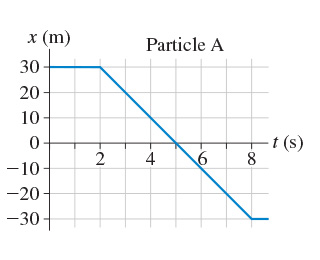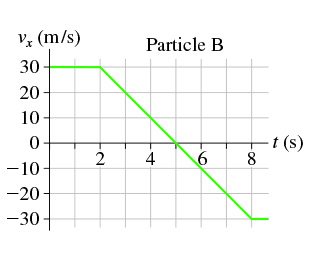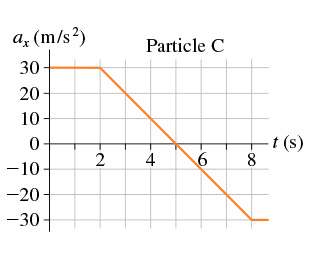# Problem: Three particles move along the x-axis, each starting at t0 = 0 s. The graph for A is a position-versus-time graph; the graph for B is a velocity-versus-time graph; the graph for C is an acceleration-versus-time graph.(a) Find the velocity of the particle A at exttip{t}{t} = 5.5 s. (b) Find the velocity of the particle B at exttip{t}{t} = 5.5 s. (c) Find the velocity of the particle C at exttip{t}{t} = 5.5 s. Particle starts with v0x = 10 m/s at t0 = 0 s.

###### FREE Expert Solution

In this problem, we have position-time, velocity-time, and acceleration-time graphs. Let's check out how to determine the velocity at a given time from each graph.

(a)

In a position-time graph, velocity is given by the slope of the curve.

80% (150 ratings)###### Problem Details

Three particles move along the x-axis, each starting at t0 = 0 s. The graph for A is a position-versus-time graph; the graph for B is a velocity-versus-time graph; the graph for C is an acceleration-versus-time graph.

(a) Find the velocity of the particle A at = 5.5 s.(b) Find the velocity of the particle B at = 5.5 s.(c) Find the velocity of the particle C at = 5.5 s. Particle starts with v0x = 10 m/s at t0 = 0 s.Frequently Asked Questions

What scientific concept do you need to know in order to solve this problem?

Our tutors have indicated that to solve this problem you will need to apply the Calculating Change in Velocity from Acceleration-Time Graphs concept. You can view video lessons to learn Calculating Change in Velocity from Acceleration-Time Graphs. Or if you need more Calculating Change in Velocity from Acceleration-Time Graphs practice, you can also practice Calculating Change in Velocity from Acceleration-Time Graphs practice problems.

What professor is this problem relevant for?

Based on our data, we think this problem is relevant for Professor Hayden's class at MSU.